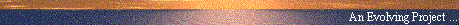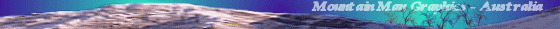# Why does Gravity Attract?

#### Barry Mingst (sci.physics)

##### Web Publication by Mountain Man Graphics, Australia# Why does Gravity Attract?

Date: 28 May 1998 00:05:47 GMT
From: Barry Mingst by way of pstowe@ix.netcom.com(Paul Stowe)
Newsgroups: sci.physics.relativity
Subject: Why does gravity attract?

```

Paul Stowe (pstowe@ix.netcom.com) wrote:

Newtonian gravity does not 'predict' instantaneous transmission,
it's an assumption that is not part of the equation.  Where in
gotten the precession of Mercury by removing this assumption
from Newton's correlation in 1898, 17 years before GR.

carlip@dirac.ucdavis.edu (Steve Carlip) writes:

This is off the main line of this thread, but I don't want to
let this claim pass, especially since Gerber's work is obscure
enough that most readers aren't going to know what to make of
this.  Paul Stowe's statement here is not correct.

First, there are two natural ways to put in "noninstantaneous"
or "retarded" transmission of gravity in Newton's equations.
One is to start with the form F=GMm/r^2 and to specify that
r is the time-delayed ("retarded") position of the source.
This leads to a loss of conservation of energy and angular
momentum and a drastic disagreement with observation---in
such a model, the Earth's orbit spirals out from the Sun,
and would have been located at the Sun's surface in historical
times.  (See exercise 12.4 in Lightman et al., _Problem Book
in Relativity and Gravitation_.)

The second is to start instead with Laplace's equation for the
gravitational potential and to change the Laplacian to a
d'Alembertian, that is, to require that the potential propagate
at the speed of light.  This also gives the wrong precession
seconds per century rather than an advance of 43 seconds.  (See
exercise 20.8 of Lightman et al.)

What Gerber did was to simply assume a rather arbitrary extra
velocity dependence of the Newtonian potential---that is, not
just that the potential depended on the retarded position, but
that it depended in a rather peculiar manner on the velocity of
the source.  There seems to have been no particular physical
justification for this dependence---von Laue concluded that Gerber
probably worked backward from the answer, and Pauli characterized
it as "completely unsuccessful from the theoretical point of view."
In any case, it was not surprising that *someone* came up with a
formula that worked.  With the discovery that the electromagnetic
potential had a velocity dependence, it was natural to guess that
the same might be true for gravity, and a dozen or so different
functional forms were tried by various physicists (none with any
particular physical justification).  Gerber was lucky enough to
come up with the combination that, in retrospect, we can recognize
as the weak field approximation of general relativity.

It is also worth mentioning that Gerber's expression for the
gravitational potential predicts a substantially wrong (and
observationally excluded) deflection of light in a gravitational
field.

Roseveare's book, _Mercury's Perihelion from Le Verrier to Einstein_.
In the meantime, rest assured that the advance of Mercury's perihelion
is most certainly not an effect of the finite propagation speed of
gravity.

Paul Stowe (pstowe@ix.netcom.com) wrote:

To get another considerd opinion on this and the related topics that
through this, the 'pseudo' treads and to prove his input.  Since he
hasn't gotten 'write' ability to the NG straightened out yet he asked
me to post his reply.  He it is:

Barry Mingst wrote:

My apologies for not responding directly.  My "reply" authority has not
yet come in.

I would like to address the subject of relativity, aether, and
fictitious forces as tied into the thread of "why does gravity
attract?"  Messrs Fischer, Stowe, Carlip, Meron and others address
various issues and make certain claims.

1) Relativity and Newton:

The relativistic principle is part of Newton's mechanics.  This
principle states that it doesn't matter what reference frame you use to
measure and interaction.  The outcome of the interaction is depends
only on the two bodies themselves.  This was a revolutionary idea at
the time (though Galileo's World Systems concept began the process).

The only catch is -- you can't use an accelerating reference frame in
Newton's mechanics without fudging the math.  As soon as you use an
accelerating reference frame for Newton's laws, you have to use
"fictitious" forces to make the equations work.  An alternate method
would be to use a "space" curvature to build in a "tendency" for matter
to deviate in predicted ways from Newtonian mechanics without a force.

2) Relativity and Maxwell:

Maxwell's equations retain the relativistic principle from Newton.  If
one examines Maxwell's equations, one finds that the electromagnetic
interactions between two matter/energy structures depend solely on the
motion and composition of the two structures.  Nothing in between has
anything to do with it (including the EM "field").

3) Relativity and Einstein:

Einstein's variation on Newtonian relativity (special relativity) is
the reaffirmation that measurable physical quantities between two
matter/energy bodies were governed solely by their relative velocities.
They were not dependent on the motion of anything in between.  This
was extrapolated from Maxwell's equations (which showed this for
electromagnetic phenomena).  Special relativity is also limited to
non-accelerating reference frames.

4) Accelerated Frames and Relativity:

If we choose any non-accelerating frame in any of the above situations,
the mechanics works out.  F=ma and all that.  But if we select an
accelerating reference frame, the bodies appear to accelerate without
our applying a force!  Now in order to describe the actions of the
bodies, we also have to take into account the accelerations of our
reference frame.

In order to "correct" the equations, we usually describe a "fictitious
force" to make the dynamics come out right.  The two fictitious forces
we normally use are centrifugal (disk rotation) and coriolis (sphere
rotation) forces.

These two forces do not exist in nature.  They have nothing to do with
matter or energy.  They are an artifact of our own human desire to use
an accelerating frame of reference for our mathematical calculations --
but to use it as if it were not accelerating.  Both of these "forces"
are pure inertia in the real world.  The "need" for coriolis forces in
modelling a flat earth is one of the strongest proofs that the earth
really isn't flat.  If you model the earth as a rotating sphere, then
there is no need for a coriolis force (but the math's harder).

For coriolis force, we could just as easily say that the surface of the
earth has an additional "sphere-time curvature".  Moving objects will
appear to accelerate along this curvature without experiencing a true
force.  Either explanation (fictitious force or curvature) makes the
answer come out right -- but both are the wrong base cause of the
observed phenomena.

5) Newtonian Gravity:

Newton's gravitational force equation is an empirical relation as to
what the force must be if the planets are to orbit in their observed
fashion.  There was and is no underlying theory of gravity or speed of
propagation in F=GMM/d^2.  This is the force equation that makes
Kepler's third law out of Newton's three laws of motion.

Functionally, this requires the speed of propagation of gravity to be
much, much higher than the orbital speeds of the planets (or you don't
get Kepler's third law).  It does not require infinite propagation.
There is no difference between "inertial" or "gravitational" mass in
Newton's equation.  G is an experimental constant that works only on
"inertial" mass (or you don't get Kepler's law).  If there is a
difference and a ratio between the two, the difference would be
buried in G anyway and we'd never know.

6) Adding Finite Propagation Speed to Newton's Gravity Equation:

There are many ways to add finite propagation to Newton's empirical
equation.  Dr. Carlip mentioned two examples of at least seven major
attempts (that I know of) prior to General Relativity.  The Ansatze of
Lorentz and others have no measurable differences in observed results
from GR.  Dr. Carlip's examples did, however, point the two major
approaches:  modify the Newtonian force equation or modify the
gravitational "field" potential equation (Laplacian, etc).

It's not a problem that Gerber's 1898 try worked backwards to get a
"right" equation (and wrong cause?).  Newton did the same.  So did
Plank to create quantum mechanics.  So did Einstein to get GR.  If you
don't have an equation, finding the source is tremendously difficult.
Maxwell's equations were one of the very rare derivations from first
principles (even though those first principles were Hemholtz' "aether
vortices").  It's also not surprising that there were unsuccessful

7) General Relativity and Accelerating Reference Frames:

General Relativity (GR) was Einstein's attempt to expand into
accelerating reference frames.  If everything gravitates, then
everything is in an accelerating state.

Contrary to Dr. Carlip's statement, GR does use a finite gravitational
propagation speed to give the precession of Mercury's orbit.  That this
does not give rise to an increase in orbital speed is a result of the
"feedback" built into the matrix of GR equations.

GR states that one can represent a "curvature" to space-time that
results in apparent (not true) acceleration.  GR interprets gravity as
a third "fictitious" force.  The key is that so long as the answer
comes out right, we can't tell whether it's s fictitious force with
curvature or a real force without curvature.  The math can't tell us.
GR was backfit onto Newton's force equation (for sticklers, the
expanded gravitational "field" potential) to get the right answers --
that's the "8 pi" part of GR.

Just like Newton's equation, GR gives no theoretical "cause" for
gravity.  There is no explanation of why space is curved by mass.  Or a
derivation of the propagational speed of space-time curvature
(postulated as c).  Or a derivation of why objects follow the shortest
"geodesics" without any external force.

And so long as the answer comes out right why the acrimony?  GR can't
tell you why it works -- it's still empirical.  Use the Ansatze of
Lorentz, Minkowski, Sommerfeld, or Mie if you like (the answers are the
same as GR).  Or try your own hand at creating something new.  No one
has succeeded in unifying gravity with the other forces yet.

The "graviton" theories of gravity are not ridiculed.  But these appear
to explicitly contradict General Relativity.  This is because gravitons
would create a "real" force, instead of a fictitious one.  According to
GR, no force is tolerable (if it REALLY is warping of space).

Barry Mingst
May 1998
```Usenet Index | Theories of Aether | Mountain Man Graphics | E-Mail